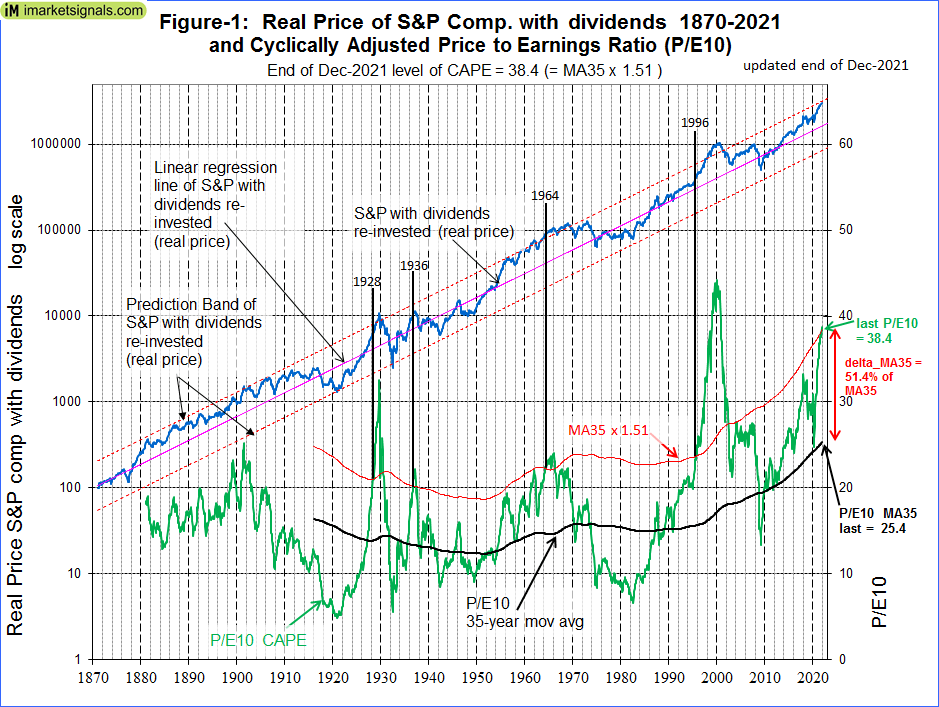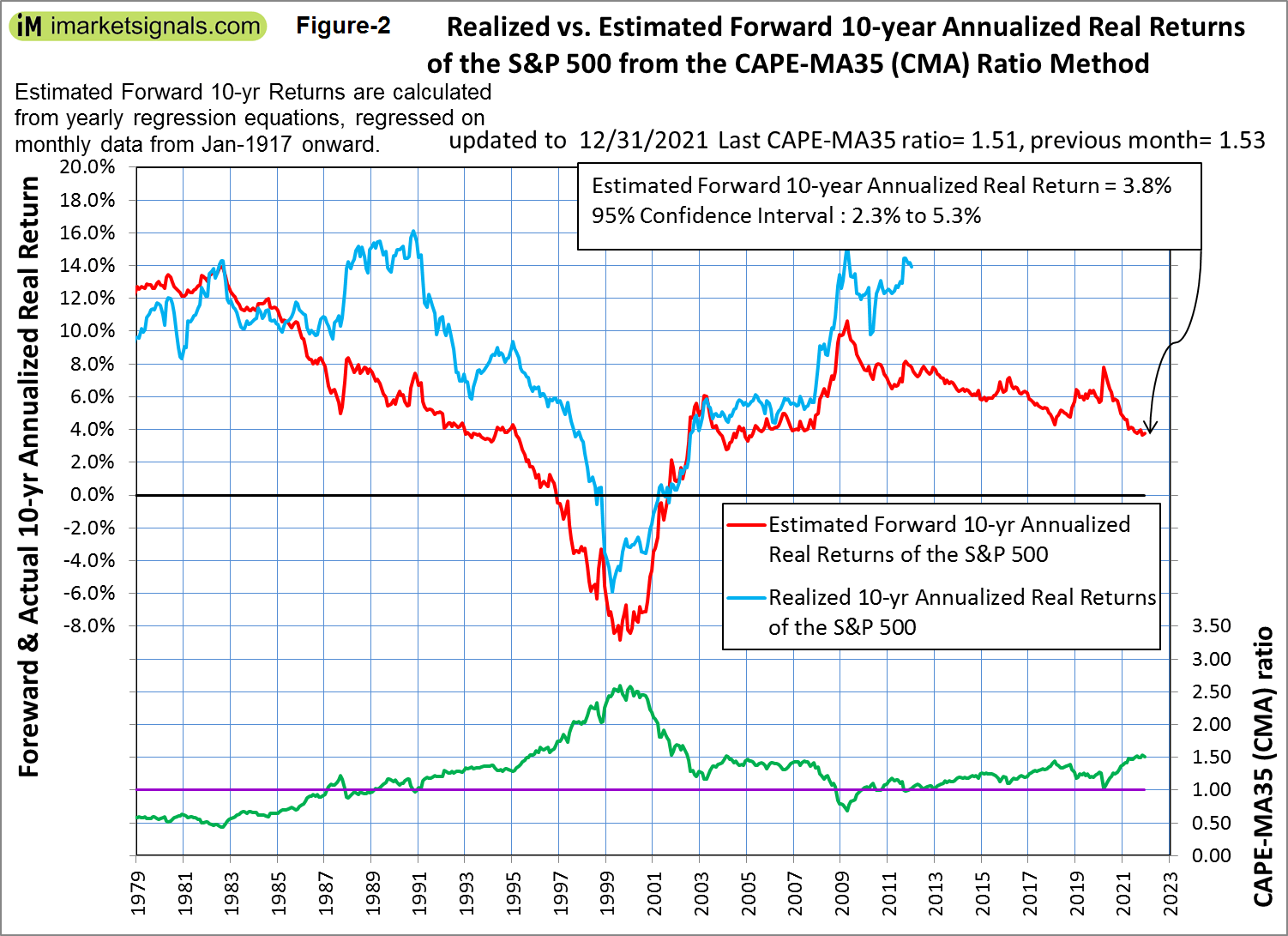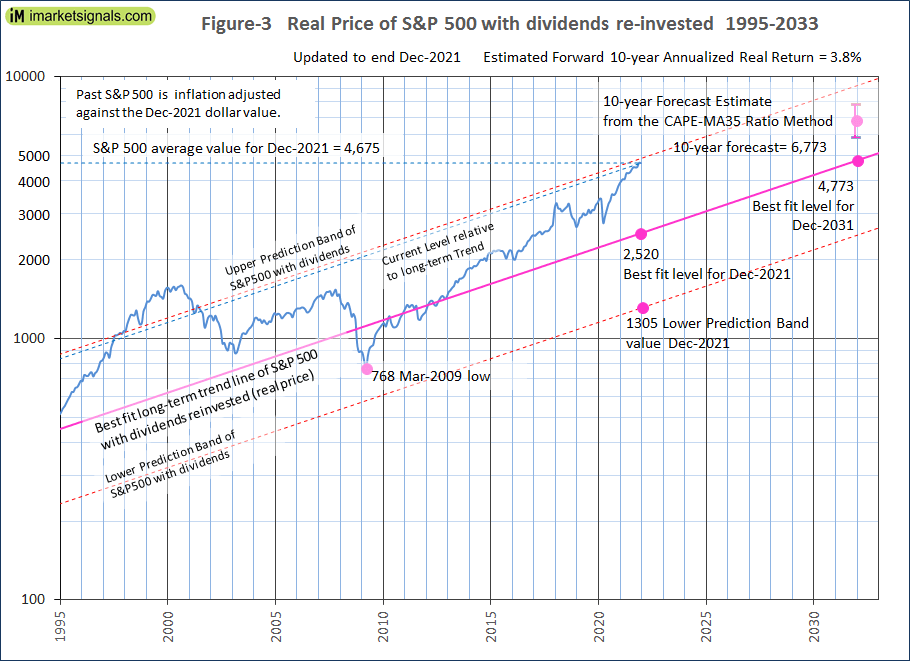# The Stock Market Is Overpriced, Expect Very Low 10-Year Forward Returns And Zero Real Returns By 2028: Update December 2021

• The average of S&P 500 for December 2021 was 4675 (previous month 4670). This is 2155 points higher than the long-term trend value of 2520.
• The current percentage difference of S&P 500 level relative to the current long-term trend level is 85%, a value not exceeded in the recent past since 2001.
• The Shiller Cyclically Adjusted Price to Earnings Ratio (CAPE) is at a level of 38.4. That is 51% higher than its 35-year moving average (MA35), currently at 25.4.
• The CAPE-MA35 ratio is 1.51, forecasting a 10-year annualized real return of about 3.8%. Should the CAPE-MA35 ratio increase further, then 10-year forward returns will be even lower.
• The historic long-term trend indicates a 10-year forward real annualized return of only 0.2%, and the current condition of overvaluation suggest zero returns by the end of 2028.

This is an update to our series of articles started in 2012 assessing market valuation and estimating forward stock market returns. The most recent previous update was for end of July 2021.

The projections here are partly based on Estimating Forward 10-Year Stock Market Returns using the Shiller CAPE Ratio and its 35-Year Moving Average, which is referred to as the “referenced article” further down.

### What short-term stock market returns can be expected?

Nobody knows, and the best one can do is to use the historic data (which is from Shiller’s S&P series) to guide us to make estimates for the future. From the real price of the S&P-Composite with dividends re-invested (S&P-real) one finds that the best-fit line from 1871 onward is a straight line when plotted to a semi-log scale. There is no reason to believe that this long-term trend of S&P-real will be interrupted. S&P-real, updated to end of December 2021 and the best fit line together with its 95% prediction band are shown in Figure-1. (See appendix for the equation.)

The current level of the S&P-real is 85% above the long-term trend line. A reversal to the mean trend would entail a 46% decline, possibly over a short period. But it is also possible if the upward earnings trend of the S&P 500 continues that S&P-real could reach the upper prediction band line in a few months. This would amount to a small gain from the current level.(click to enlarge)

### Forecasting stock market returns with the CAPE-MA35 Ratio

Also shown in Figure-1 are the CAPE (which is the S&P-real divided by the average of the real earnings over the preceding 10 years) and its 35-year moving average (MA35), having end of Dec 2021 values of 38.4 and 25.4, respectively. Thus the rising CAPE is 51% above the MA35 with the S&P-real close to the upper prediction band line.

Similar conditions of overvaluation (S&P-real near upper prediction band line and the CAPE moving above MA35*1.51) occurred only three times since 1916, in July 1928, October 1936, and March 1964. The average time to break even after those dates was 7.25 years as shown below. (In 1996 the level of S&P-real was close to the long term trend line, signaling fair valuation.)

 Period Total Return of S&P-real: July 1928 to July 1935  (7 years) 2.5% October 1936 to January 1945  (8.25 years) 1.3% March 1964 to September 1970  (6.5 years) 1.7%

As shown in the referenced article, a superior method to the standard use of the Shiller CAPE-ratio is to predict 10-year real returns using the CAPE-MA35 ratio as a valuation measure. It is simply the value of the Shiller CAPE-ratio divided by the corresponding value of its 35-year moving average (38.4 / 25.4 = 1.51).

Currently the CAPE-MA35 ratio of 1.51 forecasts a probable 10-year annualized real return of about 3.8% to December 2031. Based on the current S&P 500 value this would indicate an end of December 2031 value of 6766 for S&P-real, with upper and lower confidence values of 7830 and 5830, respectively, all as shown in Figures-2 and -3.

Also, in Figure-3 the current level of the S&P 500 relative to the long-term trend is indicated by the line parallel to the long-term trend line. Note that since mid-2001 this relative level has never been greater than what it is now.(click to enlarge)(click to enlarge)

### Forecasting returns to 2031 with the long-term trend

When extending the best fit line and the prediction bands (Figure-3), then the S&P-real end of December 2031 value would be 4773, while the highest and lowest values shown by the prediction bands would be about 9220 and 2470, respectively.

Thus, the historic trend forecasts a probable total gain of only about 2% (or an annualized return of 0.2%) for S&P-real from its current level of 4665 to the end of December 2031 best fit level of 4773. The worst-case scenario would be a possible loss of about 47% (annualized return -6.2%), and the best outcome could be a 98% gain (annualized return 7.0%).

### Conclusion

• The current elevated level of the S&P 500 relative to the current long-term trend level warns that stocks are overvalued; and
• The current CAPE-MA35 ratio of 1.51 forecasts only a 3.8% real 10-year forward return for the S&P 500, while the long-term trend indicates a forward return of only 0.2%; and
• The current condition of overvaluation suggests zero returns by the end of 2028 (7 years) based on past history when similar conditions prevailed.

One has to monitor the CAPE-MA35 ratio, because the 10-year forward returns will get lower with increasing values of this ratio. Extreme overvaluation is signaled when the CAPE-MA35 ratio exceeds a value of 1.50, which is the current value.

Updates of the estimated 10-year forward returns of the S&P 500 (Figure-2) are posted monthly at imarketsignals.com.

### Appendix

The best fit line and prediction band

The best fit line and prediction band were calculated from monthly data from Jan-1871 to July-2012.  The SP-real values for the period after July-2012 are “out of sample” and were not included in the regression analysis.

The equation of the best fit line is y = 10(ax+b)

y = is the dependent variable of the best fit line.
x = are the number of months from January 1871 onwards.
a = 0.0023112648
b = 2.02423522

Posted in 2020, blogs, featured
With reference to Section 202(a)(11)(D) of the Investment Advisers Act: We are Engineers and not Investment Advisers, read more ...